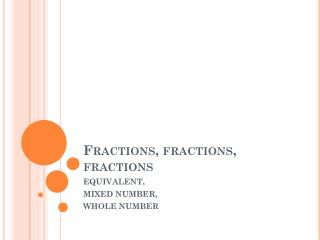DownloadDownload PresentationFractions, fractions, fractions

# Fractions, fractions, fractions

Télécharger la présentation## Fractions, fractions, fractions

- - - - - - - - - - - - - - - - - - - - - - - - - - - E N D - - - - - - - - - - - - - - - - - - - - - - - - - - -
##### Presentation Transcript

1. Fractions, fractions, fractions EQUIVALENT, MIXED NUMBER, WHOLE NUMBER

2. 2 warmups before we get started 1.) Add these fractions: 3 + 8 12 12 2.) Write what fraction this is:

3. Now lets kick it up a notch. • Fasten your seatbelts!

4. Equivalent fractions It is important to remember that EQUIVALENT means EQUAL!!! That means that two different fractions will actually be the exact same size.

5. Example In your group, find your blue bar that says 1/2 Next, find your purple bars that say 1/10 Line up your purple bars over top of the blue bar. How many purples does it take to cover the blue bar?

6. It takes 5 of the 1/10 bars to equal ½ In other words, 1/10+1/10+1/10+1/10+1/10 = 5/10 And…we know that is the same size as the 1/2 bar AND SO… 5/10 is equal to 1/2!

7. Try again! Find an orange bar that says 1/3 Next, find your black bars that say 1/12 How many black bars are equal to 1/3? Problem: ?/12 = 1/3 (How many twelfths equal a third?)

8. It should take 4 black bars to cover the orange. • So, what fraction is equal to 1/3?

9. Playtime! I will give your groups 5 minutes to explore with your fraction bars. I want you to write down as many equivalent fractions as you can!

10. Results---Tell me your equivalent fractions!

11. Now the confusing parts If you see a fraction where all the spaces are shaded in… OR You see a fraction where the top and bottom number are the same number… 4 4 That fraction = 1

12. examples 7/7 =1 =1 =1 1 2 1 2

13. Weird Fractions I lied to you before! Sometimes in fractions the big number can be on top! Let’s explore this…

14. When can this happen? When you have many shapes, and some are filled in all the way. Top number: Count every part that is shaded in. Bottom number: Count every part from ONLY ONE SHAPE How many parts are shaded?? How many parts from one of the shapes?? The fraction is:

15. Lets try that again! Top number: Count every part that is shaded in. Bottom number: Count every part from ONLY ONE SHAPE What is this fraction?

16. Practice 1.) Write a fraction that is equivalent to 1/4. 2.) Write a fraction that is equivalent to 7/7 3.) Write a fraction greater than 1/3. 4.) Write a fraction greater than 1. 5.) Draw a figure that shows the fraction 5/10. 6.) Draw a figure that shows the fraction 10/5.